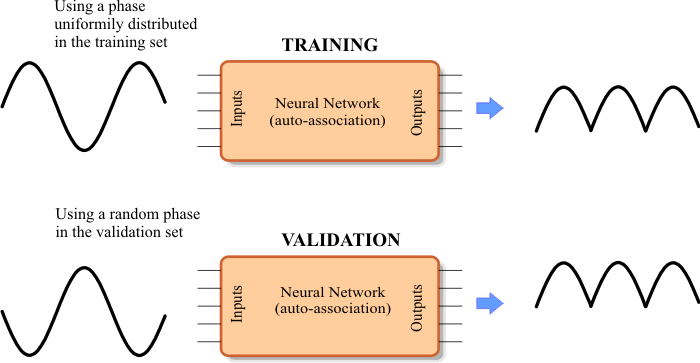# Full Wave Rectifier

 Problem 1 Create a New Project called Rectifier to build an appropriate training set to use an ANN to rectify a sine wave as shown in the figure. Use a uniformly distributed phase for each training case.Problem 2 Edit the BuilValidSet.lab file to build the validation set using random phase for each validation case.

 Problem 3 Edit the Train.lab file to design and train an ANN to rectify a sine wave. Train the ANN using simulated annealing and the conjugate gradient method.

 Problem 4 Edit the CheckTraining.lab file to check the training: (a) Compute the mean squared error for the ANN using the training set. (b) Plot the error for each training case. (c) Save the plot as a vector image (checkTraining.pdf and checkTraining.emf)

 Problem 5 Edit the Validation.lab file to perform the validation of the ANN. (a) Compute the mean squared error for the ANN using the validation set. (b) Plot the error for each validation case. (c) Save the plot as a vector image (validation.pdf and validation.emf)

 Problem 6 Generate a report in Microsoft Word. Write some conclusions in the report focusing on the problems that were faced during the simulation and how these problems were or could be solved.